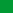Db2 11.5

# Examples of subselect queries with joins

The following examples illustrate the use of joins in a subselect query.

• Example 1:  This example illustrates the results of the various joins using tables J1 and J2. These tables contain rows as shown.
``````   SELECT * FROM J1

W   X
--- ------
A       11
B       12
C       13

SELECT * FROM J2

Y   Z
--- ------
A       21
C       22
D       23``````

The following query does an inner join of J1 and J2 matching the first column of both tables.

``````   SELECT * FROM J1 INNER JOIN J2 ON W=Y

W   X      Y   Z
--- ------ --- ------
A       11 A       21
C       13 C       22``````

In this inner join example the row with column W='C' from J1 and the row with column Y='D' from J2 are not included in the result because they do not have a match in the other table. Note that the following alternative form of an inner join query produces the same result.

``   SELECT * FROM J1, J2 WHERE W=Y``

The following left outer join will get back the missing row from J1 with nulls for the columns of J2. Every row from J1 is included.

``````   SELECT * FROM J1 LEFT OUTER JOIN J2 ON W=Y

W   X      Y   Z
--- ------ --- ------
A       11 A       21
B       12 -        -
C       13 C       22``````

The following right outer join will get back the missing row from J2 with nulls for the columns of J1. Every row from J2 is included.

``````   SELECT * FROM J1 RIGHT OUTER JOIN J2 ON W=Y

W   X      Y   Z
--- ------ --- ------
A       11 A       21
C       13 C       22
-        - D       23``````

The following full outer join will get back the missing rows from both J1 and J2 with nulls where appropriate. Every row from both J1 and J2 is included.

``````   SELECT * FROM J1 FULL OUTER JOIN J2 ON W=Y

W   X      Y   Z
--- ------ --- ------
A       11 A       21
C       13 C       22
-        - D       23
B       12 -        -``````
• Example 2:  Using the tables J1 and J2 from the previous example, examine what happens when and additional predicate is added to the search condition.
``````   SELECT * FROM J1 INNER JOIN J2 ON W=Y AND X=13

W   X      Y   Z
--- ------ --- ------
C       13 C       22``````

The additional condition caused the inner join to select only 1 row compared to the inner join in Example 1.

Notice what the affect of this is on the full outer join.

``````   SELECT * FROM J1 FULL OUTER JOIN J2 ON W=Y AND X=13

W   X      Y   Z
--- ------ --- ------
-        - A       21
C       13 C       22
-        - D       23
A       11 -        -
B       12 -        -``````

The result now has 5 rows (compared to 4 without the additional predicate) because there was only 1 row in the inner join and all rows of both tables must be returned.

The following query illustrates that placing the same additional predicate in WHERE clause has completely different results.

``````   SELECT * FROM J1 FULL OUTER JOIN J2 ON W=Y
WHERE X=13

W   X      Y   Z
--- ------ --- ------
C       13 C       22``````

The WHERE clause is applied after the intermediate result of the full outer join. This intermediate result is the same as the result of the full outer join query in Example 1. The WHERE clause is applied to this intermediate result and eliminates all but the row that has X=13. Choosing the location of a predicate when performing outer joins can have a significant affect on the results. Consider what happens if the predicate was X=12 instead of X=13. The following inner join returns no rows.

``   SELECT * FROM J1 INNER JOIN J2 ON W=Y AND X=12``

Hence, the full outer join returns 6 rows, 3 from J1 with nulls for the columns of J2 and 3 from J2 with nulls for the columns of J1.

``````   SELECT * FROM J1 FULL OUTER JOIN J2 ON W=Y AND X=12

W   X      Y   Z
--- ------ --- ------
-        - A       21
-        - C       22
-        - D       23
A       11 -        -
B       12 -        -
C       13 -        -``````

If the additional predicate is in the WHERE clause instead, 1 row is returned.

``````   SELECT * FROM J1 FULL OUTER JOIN J2 ON W=Y
WHERE X=12

W   X      Y   Z
--- ------ --- ------
B       12 -        -``````
• Example 3:  List every department with the employee number and last name of the manager, including departments without a manager.
``````  SELECT DEPTNO, DEPTNAME, EMPNO, LASTNAME
FROM DEPARTMENT LEFT OUTER JOIN EMPLOYEE
ON MGRNO = EMPNO``````
• Example 4:  List every employee number and last name with the employee number and last name of their manager, including employees without a manager.
``````  SELECT E.EMPNO, E.LASTNAME, M.EMPNO, M.LASTNAME
FROM EMPLOYEE E LEFT OUTER JOIN
DEPARTMENT INNER JOIN EMPLOYEE M
ON MGRNO = M.EMPNO
ON E.WORKDEPT = DEPTNO``````

The inner join determines the last name for any manager identified in the DEPARTMENT table and the left outer join guarantees that each employee is listed even if a corresponding department is not found in DEPARTMENT.